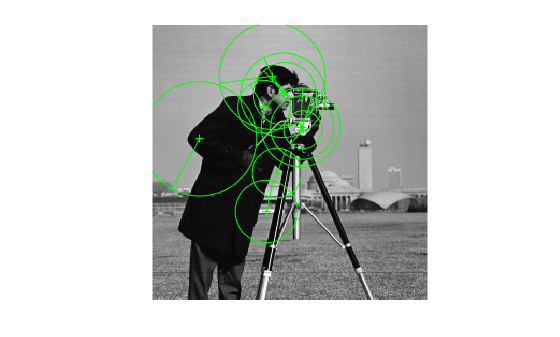# isempty

Determine if points object is empty

## Syntax

``isempty(points)``

## Description

example

````isempty(points)` returns a `true` value, if the points object is empty.```

## Examples

collapse all

`I = imread('cameraman.tif');`

Detect KAZE points from the image.

`points = detectKAZEFeatures(I);`

Check for points.

```if isempty(points) return; end```

Extract KAZE features from the detected points.

`[features,validPoints] = extractFeatures(I,points);`

Plot the ten strongest valid extracted points and show their orientations.

```imshow(I) hold on strongestPoints = selectStrongest(validPoints,10); plot(strongestPoints,'showOrientation',true) hold off```## Input Arguments

collapse all

Points object,specified as an M-by-2 matrix of M number of [x y] coordinates, or as one of the point feature objects described in Point Feature Types. The object contains information about the feature points detected in the input image. To obtain points, use the appropriate detect function that pairs with the point feature type.

## Version History

Introduced in R2017b